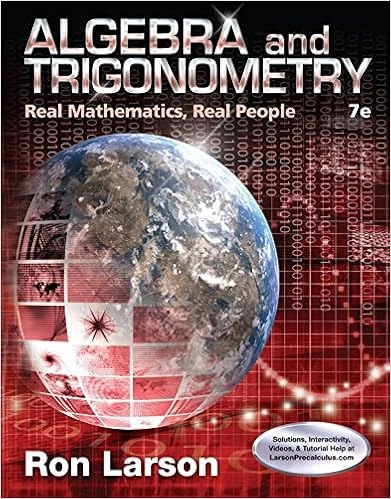# D the table below shows the energies of the simple

• Homework Help
• 12
• 100% (1) 1 out of 1 people found this document helpful

This preview shows page 10 - 12 out of 12 pages.

##### We have textbook solutions for you!
The document you are viewing contains questions related to this textbook.The document you are viewing contains questions related to this textbook.
Chapter 5 / Exercise 70
Algebra and Trigonometry: Real Mathematics, Real People
LarsonExpert Verified
(d) The table below shows the energies of the simple harmonic motion. Complete the table. gravitational potential energy/J elastic potential energy/J kinetic energy/J total energy/J lowest point equilibrium position highest point (e) On the axes of diagram below, sketch four graphs to show the shape of the variation with position of the four energies. Label each graph. [0.785 J, 0.392 J, 3.92 N, 7.00 rad s -1 , 1.40 m s -1 , total energy = 1.57 J] energy/J lowest point equilibrium position highest point 0
##### We have textbook solutions for you!
The document you are viewing contains questions related to this textbook.The document you are viewing contains questions related to this textbook.
Chapter 5 / Exercise 70
Algebra and Trigonometry: Real Mathematics, Real People
LarsonExpert Verified
2016 Temasek Junior College 11 (a) Frequency is the number of oscillations per unit time and its unit is Hz. Angular frequency is the product of 2 and frequency and it has a unit of rad s -1 . (b) (i) Loss in GPE = mge = 0.400 (9.81)0.200 = 0.785 J (ii) 1 1 1 Gain in EPE = 0.400 9.81 0.200 0.392 J 2 2 2 Te mge The difference is the negative work done by the hand exerting an upward force to gently lower the mass. (c) (i) At lowest point, total extension = 2e 2 = 2 2 mg T k e e mg e + Resultant force on the load = 2mg mg = mg = 0.400 (9.81) = 3.92 N upwards (ii) o x m ma F 2 2 -1 3.92 0.400 0.200 7.00 rad s (iii) o x v max -1 s m 40 . 1 200 . 0 00 . 7 (d) gravitational potential energy/J elastic potential energy/J kinetic energy/J total energy/J lowest point 0 1.57 0 1.57 equilibrium position 0.785 0.392 0.392 1.57 highest point 1.57 0 0 1.57 (e)
19. 2013P3Q7 (part) (a) A tube, sealed at one end, has a uniform area of cross-section A . Some sand is placed in the tube so that it floats upright in a liquid of density as shown in the figure. The total mass of the tube and the sand is m The tube floats with its base a distance h below the surface of the liquid. Derive an expression relating m to h, A and . Explain your working. (b) The tube is displaced vertically and then released. For a displacement x , the acceleration a of the tube is given by the expression Ag a x m   where g is the acceleration of the free fall. (i) Explain why the expression leads to the conclusion that the tube is performing simple harmonic motion. (ii) The tube has a total mass m of 32 g and the area A of its cross-section 4.2 cm 2 . It is floating in liquid of density of 1.0 x 10 3 kg m -3 Show that the frequency of oscillation of the tube is 1.8 Hz. [1.8 Hz] (a) Since the tube is in equilibrium, net force = 0. . .
(b)(i) Since , g, A and m are constants, => a x.
2016 Temasek Junior College 12
•••